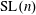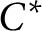Home

### Topological linear spaces and related structures (46Axx)

• Article
• ##### Interpolation between noncommutative martingale Hardy and BMO spaces: the case$\textbf{0}\boldsymbol{<}\textbf{p}\boldsymbol{<}\textbf{1}$
• Canadian Journal of Mathematics, First View

• Article
• #####$\text{SL}(n)$ Invariant Valuations on Super-Coercive Convex Functions
• Canadian Journal of Mathematics, Volume 73, Issue 1

### Normed linear spaces and Banach spaces; Banach lattices (46Bxx)

• Article
• ##### Interpolation between noncommutative martingale Hardy and BMO spaces: the case$\textbf{0}\boldsymbol{<}\textbf{p}\boldsymbol{<}\textbf{1}$
• Canadian Journal of Mathematics, First View

### Commutative Banach algebras and commutative topological algebras (46Jxx)

• Article
• #####$C^*$ -algebra structure on certain Banach algebra products
• Canadian Mathematical Bulletin, Volume 64, Issue 3

### Selfadjoint operator algebras ($C^*$-algebras, von Neumann ($W^*$-) algebras, etc.) (46Lxx)

• Article
• ##### Interpolation between noncommutative martingale Hardy and BMO spaces: the case$\textbf{0}\boldsymbol{<}\textbf{p}\boldsymbol{<}\textbf{1}$
• Canadian Journal of Mathematics, First View